(Approximate)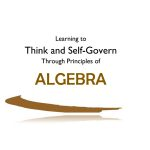Basic Algebra

(Ages 12 and up, 48 pages) The intrinsic value for math is “learning to think and self-govern using correct principles.” This student workbook contains 30 principles of algebra, and an introduction explains the basics of why algebra uses letters to represent unknown numbers. Also included is a page of 19 …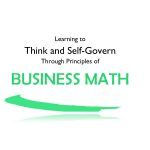(Ages 12 and up, 53 pages) The intrinsic value for math is “learning to think and self-govern using correct principles.” This student workbook contains 37 principles of business math, and an introduction gives an explanation of how “business” works in different scenarios. Dealing with money is the most common exposure …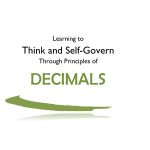Decimals

(Ages 10 and up, 26 pages) The intrinsic value for math is “learning to think and self-govern using correct principles.” This student workbook contains 19 principles of decimals, and an introduction explains the origin of the dollar sign and cents. Writing amounts of money is the most common exposure young …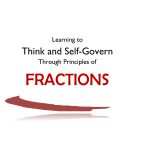Fractions

(Ages 10 and up, 38 pages) The intrinsic value for math is “learning to think and self-govern using correct principles.” This student workbook contains 33 principles of fractions, and students learn the basics of adding, subtracting, multiplying, and dividing fractions, as well as reducing. Vocabulary words students learn along with …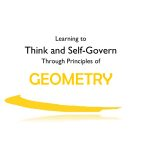Geometry

(Ages 12 and up, 52 pages) The intrinsic value for math is “learning to think and self-govern using correct principles.” This student workbook contains 34 principles of geometry, and an introduction explains the many uses of geometry in occupations and jobs. Students learn the basics of geometrical shapes, as well …Heavenly Father’s Favorite Numbers — Volume 1

Some of the basic principles taught in this volume are: Heavenly Father Counts Everything He Creates Heavenly Father Counts Every Person on Earth Heavenly Father has Favorite Numbers Heavenly Father Has Organized Numbers Into Beautiful Patterns Symbols Give LIFE to Numbers — numbers can be carried and borrowed! Story Problems …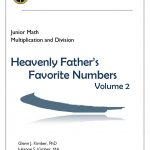Heavenly Father’s Favorite Numbers — Volume 2

This book of numeration volume 2 for young people presents basic principles of multiplication facts and division principles.  As with Volume 1, this book will help students understand that everything in the world and in the universe is patterned by God and His use of numbers. As with Volume 1, …Heavenly Father’s Favorite Numbers Volume 3–FRACTIONS

How does Heavenly Father use fractions? You will be surprised to discover many scriptures that refer to fractions — such as a “third” of the hosts of heaven, found in the book of Revelation. With this fun new addition to our junior math program, the younger students will gain a …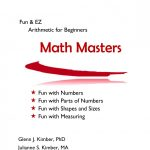Math Masters

Math Masters is written for children ages 4 to 8, with an emphasis on having FUN with numbers and their application.   (109 pages)

Numeration

(Ages 10 and up, 40 pages) The intrinsic value for math is “learning to think and self-govern using correct principles.” This student workbook contains 20 principles of numeration (whole numbers), and students learn the basics of addition, subtraction, multiplication, and division. The principles of exponents and order of operations are …Percents

(Ages 10 and up, 22 pages) The intrinsic value for math is “learning to think and self-govern using correct principles.” This student workbook contains 15 principles of percents, and an introduction explains to the student why it is important to understand how percentages work. Students learn the relationship of percents …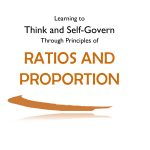Ratios and Proportions

(Ages 10 and up, 21 pages) The intrinsic value for math is “learning to think and self-govern using correct principles.” This student workbook contains 15 principles of ratio & proportion, and an introduction explains the importance of understanding these principles. Students learn various vocabulary words associated with this subject, such …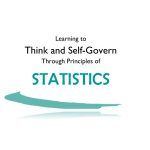Statistics

(Ages 12 and up, 26 pages) The intrinsic value for math is “learning to think and self-govern using correct principles.” This student workbook contains 15 principles of statistics. Students learn the basics of different kinds of charts which compare and analyze data. Students study and draw circle graphs, bar graphs, …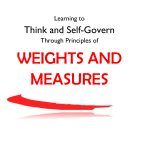Weights and Measures

(Ages 12 and up, 46 pages) The intrinsic value for math is “learning to think and self-govern using correct principles.” This student workbook contains 27 principles of weights and measures. Students learn the basic standards of weighing and measuring with the customary units used in the U.S. They also learn …